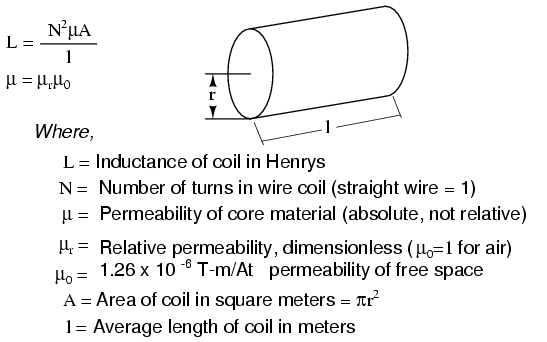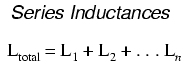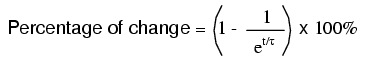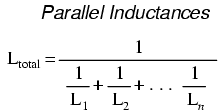# Learning Electronics

Learn to build electronic circuits

# Inductor sizing equationWheeler's formulas for inductance of air core coils which follow are usefull for radio frequency inductors. The following formula for the inductance of a single layer air core solenoid coil is accurate to approximately 1% for 2r/l < 3. The thick coil formula is 1% accurate when the denominator terms are approximately equal. Wheeler's spiral formula is 1% accurate for c>0.2r. While this is a "round wire" formula, it may still be applicable to printed circuit spiral inductors at reduced accuracy.The inductance in henries of a square printed circuit inductor is given by two formulas where p=q, and p≠q.The wire table provides "turns per inch" for enamel magnet wire for use with the inductance formulas for coils.